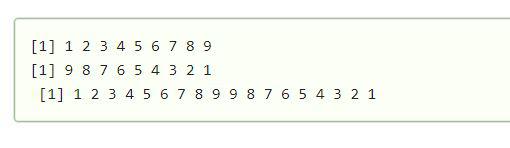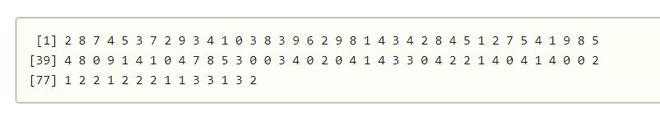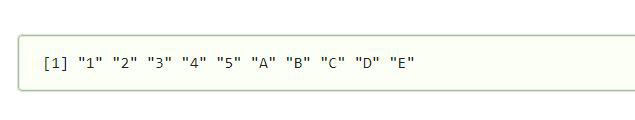# How to concatenate two or more vectors in R ?

• Last Updated : 05 Apr, 2021

In this article, we will see how to concatenate two or more vectors in R Programming Language.

To concatenate two or more vectors in r we can use the combination function in R. Let’s assume we have 3 vectors vec1, vec2, vec3 the concatenation of these vectors can be done as c(vec1, vec2, vec3). Also, we can concatenate different types of vectors at the same time using the same function.

Concatenate function:

• This is a function that combines its arguments.
• This method combines the arguments and results in a vector.
• All the arguments are converted to a common type that is the return value type.
• This function is also used to convert the array into vectors.

Syntax: c(argument, argument,..)

Parameter:

Arguments : The objects to be concatenated

Approach:

• Create a number of example vectors to concatenate.
• Concatenate the vectors by c function, c(vec1, vec2, vec3)

Example 1: Let’s first create an example vector and then concatenate those vectors.

In this example, we have first created a vector vec1 and vec2. Then by using the concatenate function we have concatenated both the vectors to get the result.

## R

 `vec1 <- 1:9``vec1`` ` `vec2 <-9:1``vec2`` ` `c``(vec1,vec2)`

Output:Example 2: Lets us now concatenate 3 vectors.

In this example, we have created 3 vectors vec1,vec2,vec3 using sample function and then concatenate the vectors into a single vector using the concatenate function

## R

 `vec1 <- ``sample``(0:9 , 50 , replace = ``TRUE``)``vec2 <- ``sample``(0:4 , 25 ,replace = ``TRUE``)``vec3 <- ``sample``(1:3 , 15 , replace = ``TRUE``)`` ` `c``(vec1,vec2,vec3)`

Output:.

Example 3: In this example, we have created a character vector and a number vector and then combine both the vectors using the concatenate function of R library.

## R

 `number <- (1, 2, 3, 4, 5)``character <- (``"A"` `, ``"B"` `, ``"C"` `, ``"D"` `, ``"E"``)`` ` `c``(number, character)`

Output:My Personal Notes arrow_drop_up